Introduction to Diﬀerential Geometry
Juan M. Bello-Rivas
bello-rivas@princeton.edu
June 7, 2017Outline
1 Basic concepts and tools
2 Connections, covariant diﬀerentiation, and parallel translation
3 CurvatureOutline
1 Basic concepts and tools
2 Connections, covariant diﬀerentiation, and parallel translation
3 Curvature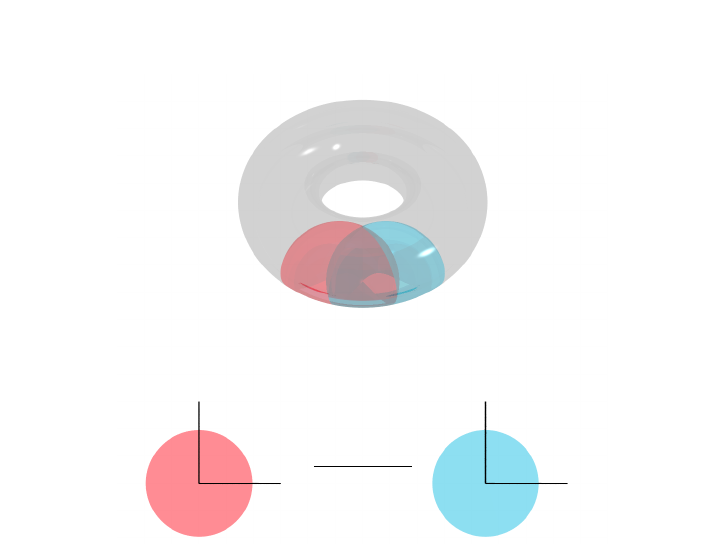Smooth manifolds
@
@
@
@R
-
ϕ ψ
ψ ϕ
1
R
m
R
m
Smooth m-dimensional manifold with two overlapping charts.Smooth maps
Deﬁnition
Let M, N be smooth manifolds and let {(U, ϕ)}, {(V, ψ)} be
atlases determining (respectively) the smooth structure of M and
N. A continuous mapping f : M N is said to be a smooth
map, if the composition ψ f ϕ
1
is of class C
.Smooth maps
A function f : M R is said to be smooth (or of class C
) if it
has “suﬃciently” many derivatives (and these are continuous).
The set of all smooth functions from M to N is denoted by
C
(M, N).
The set of all real-valued smooth functions on M is denoted by
C
(M).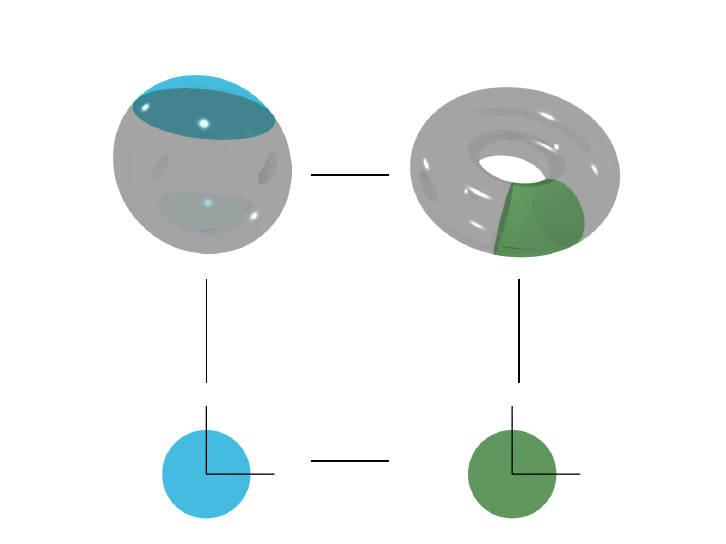Smooth maps
p
f(p)
?
ϕ
6
ψ
1
f
-
-
F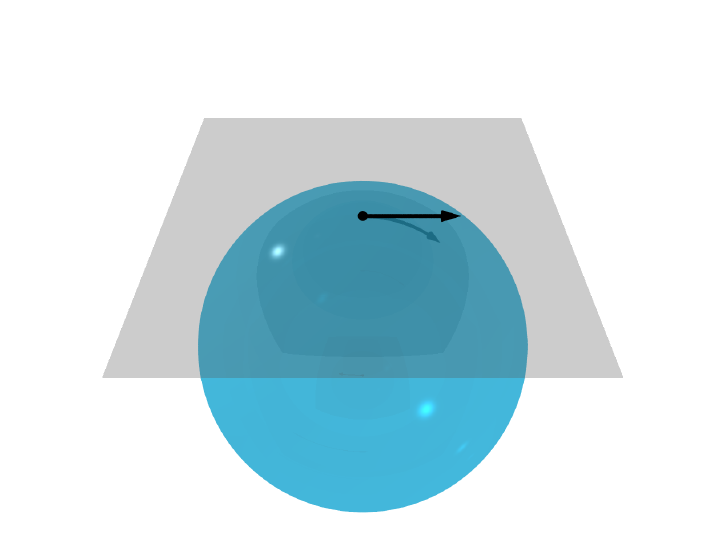Tangent vectors and tangent spaces
p
T
p
M
MTangent vectors and tangent spaces
Deﬁnition (Tangent vector)
Let M be a smooth m-dimensional manifold and let p M. A
tangent vector v at p is a map v : C
(M) R such that
1 v(f + g) = v(f) + v(g),
2 v(λf) = λv(f),
3 v(fg) = v(f) g(p) + f (p) v(g),
For any f, g C
(M) and λ R.Tangent vectors and tangent spaces
The set of tangent vectors at p M is a vector space called the
tangent space, denoted by T
p
M. Indeed, if v, w are tangent
vectors at p and λ R, then
1 (v + w)(f) = v(f) + w(f ),
2 (λ v)(f ) = λ v(f).
In a local chart, the vectors
x
1
p
, . . . ,
x
m
p
constitute a basis of T
p
M.Tangent vectors and tangent spaces
Example
Let M = R
m
. In this case, the directional derivative of
f C
(M) at p M in the direction v = (v
1
, . . . , v
m
) R
m
is
f(p) · v =
X
i
v
i
f
x
i
(p).
In our current notation, we would instead say that
v =
X
i
v
i
x
i
p
is a tangent vector of M at p.Tangent vectors and tangent spaces
We can also view each tangent vector at p as the velocity vector of
a curve γ : [0, T ] R M such that γ(0) = p. In that case,
v(f) =
d
dt
f(γ(t)) =
X
i
˙γ
i
(t)
f
x
i
(γ(t))
=
X
i
˙γ
i
(t)
|{z}
=v
i
x
i
γ(t)
(f).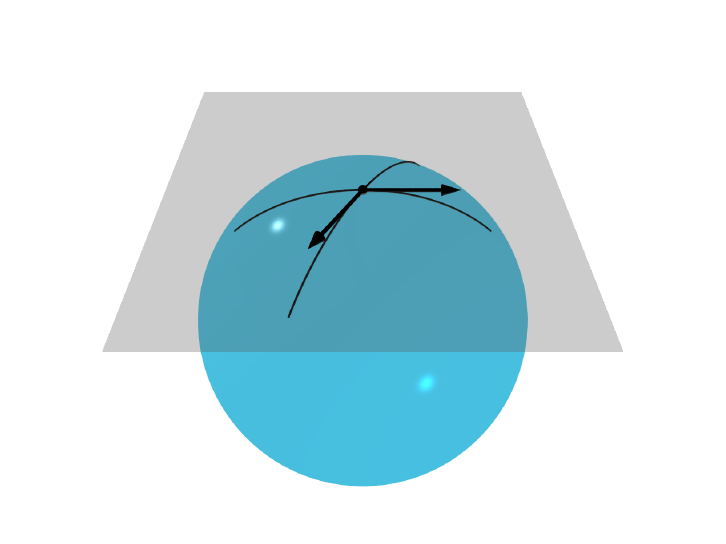Tangent vectors and tangent spaces
p
T
p
M
MVector ﬁelds
Deﬁnition (Vector ﬁeld)
A vector ﬁeld is a correspondence of a tangent vector v
p
to each
point p M. The set of all vector ﬁelds over a manifold M is
denoted by X(M ).
In local coordinates,
v
p
=
X
i
v
i
(p)
x
i
p
From now on, we adopt the convention
i
=
x
i
.Vector ﬁelds
To any smooth vector ﬁeld v X(M) and any t R, we can
associate the ﬂow map, Φ
t
: M M, that satisﬁes
d
dt
Φ
t
(p) = v
Φ
t
(p)
.
In other words, t 7→ Φ
t
(p) is an integral curve of v.
In local coordinates, if v =
P
i
v
i
i
, then
t 7→ Φ
t
(p) = (γ
1
(t), . . . , γ
m
(t)) solves the initial value problem
d
dt
γ
i
(t) = v
i
(γ(t)),
for each i = 1, . . . , m.
with initial condition Φ
0
(p) = γ(0) = p.Lie bracket
Deﬁnition (Lie bracket)
The Lie bracket of two vector ﬁelds v, w X(M ) is the map
[·, ·] : X(M ) × X(M ) X(M)
deﬁned by
(v, w) 7→ [v, w] = vw wv
Proposition
The Lie bracket of two vector ﬁelds is a linear diﬀerential operator
(the second order derivatives vanish).Lie bracket
In local coordinates,
[
i
,
j
] =
i
j
j
i
= 0.
The Lie bracket leads us to the generalization of the Theorem of
existence and uniqueness of solutions of ODEs to multiple vector
ﬁelds. This general result is known as the Frobenius Theorem.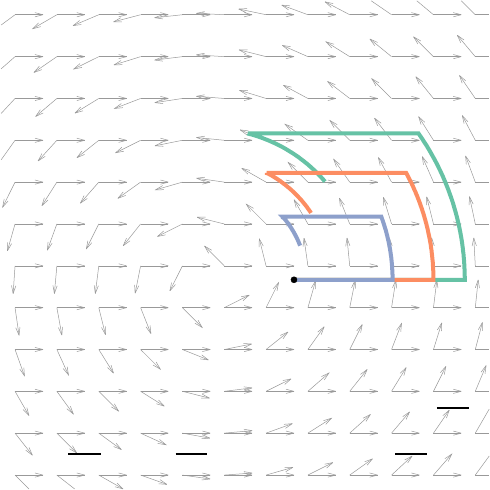x
Lie bracket (geometric interpretation)
The value of the Lie bracket of v =
x
and
w = y
x
+ x
y
is [v, w] =
y
.Push-forward
Let M and N be two smooth manifolds and let f : M N be a
smooth map.
Deﬁnition (Diﬀerential of a map)
The map
f
?
: T
p
M T
f(p)
N
deﬁned for each h C
(N) by
v T
p
M 7→ f
v(h) = v(h f) R
is a linear map called the diﬀerential map of f .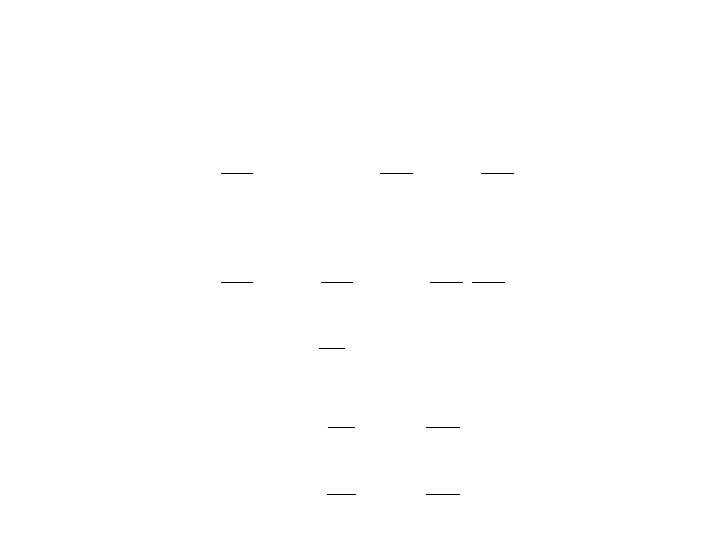Push-forward
In local coordinates (x
1
, . . . , x
m
) for M and (y
1
, . . . , y
n
) for N , we
have y
i
= f(x
1
, . . . , x
m
) and
f
?
(
x
i
)
(h) =
X
j
h
y
j
(f(x))
y
j
x
i
(x).
Therefore,
x
i
7→ f
?
(
x
i
) =
X
j
y
j
x
i
y
j
Observe that the vectors f
?
(
x
i
) for i = 1, . . . , m are the columns
of the Jacobian matrix of f,
Df =
y
1
x
1
. . .
y
1
x
m
.
.
.
.
.
.
.
.
.
y
n
x
1
. . .
y
n
x
mPush-forward
The natural application of the push-forward is the transformation
of vector ﬁelds on M into vector ﬁelds on N.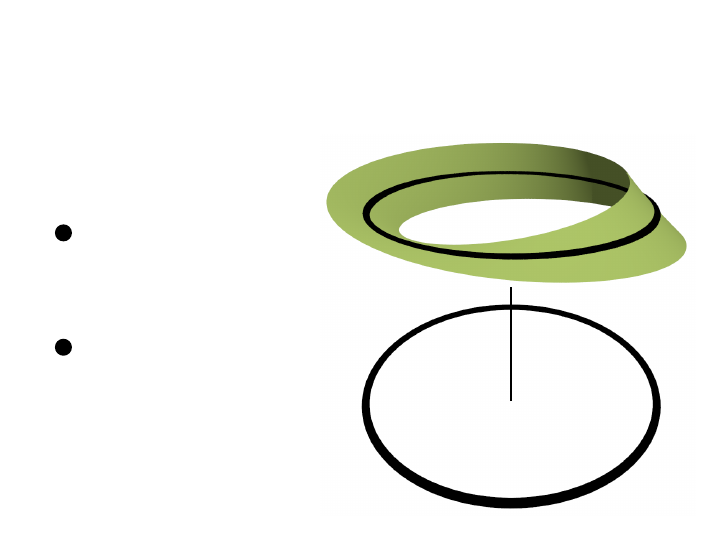Vector bundles
A vector bundle over M
is a tuple (E, π, M)
where:
1 The total space E
and the base space
M are smooth
manifolds.
2 The projection
π : E M is a
smooth map that
conditions.
?
π
M
E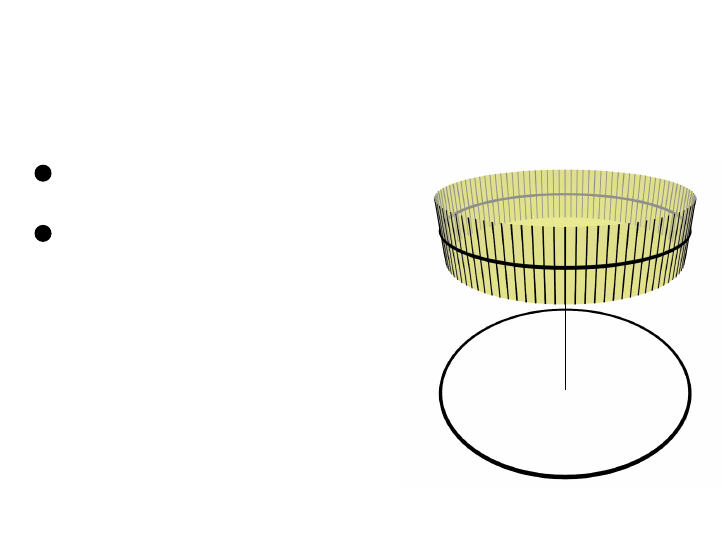Vector bundles
The projection π : E M is a smooth
map that satisﬁes the following
conditions:
1 For each p M , the ﬁber
E
p
= π
1
(p) is a vector space.
2 local triviality: For each p M,
there exists an open neighborhood
U of p and a diﬀeomorphism
ϕ
U
: π
1
(U) U × R
n
such that for each q U , we have
that
ϕ
U
|
π
1
(q)
: π
1
(q) {q} × R
n
is a linear isomorphism.
?
π
M
EVector bundles (examples)
The tangent bundle,
T M =
[
pM
T
p
M.
The cotangent bundle, denoted by T M
?
or T
?
M.
The phase space of a mechanical system.
Frenet frame of a curve.Sections
Deﬁnition
Let π : E M be a vector bundle. A section is a smooth map
s: M E
p 7→ s(p) E
p
such that π s = 1
M
. Indeed, we have the following diagram:
E
π
M
s
OO
The set of sections of a vector bundle E, denoted by Γ(E), is a
vector space with the following operations:
0
)(p) = s(p) + s
0
(p)
product (λ s)(p) = λ s(p)Diﬀerential forms
Consider local coordinates (x
1
, . . . , x
m
) in a neighborhood of the
point p M. Consider the dual space
T
p
M
?
= {ω : T
p
M R | ω is linear}
to T
p
M whose basis is
dx
1
, . . . , dx
m
T
p
M
?
such that
dx
i
(
j
) = δ
i
j
=
(
1 i = j,
0 i 6= j.
The elements of the vector space T
p
M
?
are called diﬀerential
1-forms.Diﬀerential forms
Let f C
(M). The exterior derivative of f is
df =
X
i
f
x
i
dx
i
.
If v =
P
i
v
i
i
, then
df(v) =
X
i
f
x
i
dx
i
X
j
v
j
j
=
X
i
f
x
i
v
j
= v(f) = f · v.
Discuss geometric interpretation + work along a path, etc.Pull-back
Let M and N be two smooth manifolds and let
f : M N
be a smooth map between them. The diﬀerential
f
?
: T
p
M T
f(p)
N at each p M induces a map
f
?
: T
?
f(p)
N T
?
p
M
ω 7→ f
?
ω,
such that
(f
?
ω)(v) = ω(f
?
v) = (ω f
?
)(v) for all v T
p
M.
Also, we have df
?
ω = f
?
(dω).Tensors
Tensors are elements of tensor spaces, which in turn are
particular cases of vector spaces.
Let V and W be two vector spaces. The tensor space V W of
V and W is the vector space generated by elements v w
satisfying:
(αv
1
+ βv
2
) w = αv
1
w + βv
2
w
and
v (αw
1
+ βw
2
) w = αv w
1
+ βv w
2
,
where v, v
1
, v
2
V , w, w
1
, w
2
W , and α, β R.
The tensor product is unique up to a linear isomorphism.Tensors
Covariant tensors transform via push-forwards (like tangent
vectors) and contravariant tensors transform through pull-backs
(like diﬀerential forms).
The construction of tensor spaces can be generalized to more than
two vector spaces (e.g., V
1
V
2
· · · V
k
).
A tensor space involving r contravariant vector spaces and s
covariant tensor spaces is a
r
s
-tensor space.Tensors
Example (Matrices)
We can regard the matrix
A =
A
1
1
· · · A
m
1
.
.
.
.
.
.
.
.
.
A
1
m
· · · A
m
m
,
as an element of the tensor space R
m
(R
m
)
?
. Indeed, if
R
m
= he
1
, . . . , e
m
i and (R
m
)
?
= he
1
, . . . , e
m
i, then
A =
X
ij
A
i
j
e
i
e
j
.
We say that A is an endomorphism of the vector space E and
denote that by A End(E).Riemannian metric
Deﬁnition (Riemannian metric)
Let M be an m-dimensional smooth manifold and let
g Λ
1
(T
?
M) Λ
1
(T
?
M) be such that for an open set U M
and a system of coordinates x = (x
1
, . . . , x
m
) in U, we have
g
ij
(p) = g(
i
,
j
) = h
i
,
j
i,
for all p U and i, j = 1, . . . , m. The tangent vectors
1
, . . . ,
m
T
p
M are assumed to form an orthonormal frame.
The Riemannian metric g
p
(v, w) at p M is the inner product
between the tangent vectors v, w T
p
M.Riemannian metric
The Euclidean metric in R
n
is
g =
m
X
i=1
dx
i
dx
i
. (1)
Consider the polar coordinates in R
2
given by
x
1
(r, θ) = r cos θ, x
2
(r, θ) = r sin θ,
where r 0 and 0 < θ < 2π. We have
dx
1
= cos θ dr r sin θ dθ and dx
2
= sin θ dr + r cos θ dθ.
Therefore, the Euclidean metric (1) is pulled back as:
g = dx
1
dx
1
+ dx
2
dx
2
= dr dr + r
2
dθ dθ.
While in Euclidean coordinates we have g
ij
= δ
ij
, in polar
coordinates we have g
11
= 1, g
22
= r
2
, g
12
= g
21
= 0.First fundamental form
Indeed, if g =
P
i
dz
i
dz
i
is the Euclidean metric on R
n
, then its
pullback by u 7→ z = ϕ(u) is given by:
ϕ
?
g =
X
i
ϕ
?
dz
i
ϕ
?
dz
i
=
X
i
X
j
ϕ
i
u
j
du
j
X
k
ϕ
i
u
k
du
k
!
=
X
j
X
k
X
i
ϕ
i
u
j
ϕ
i
u
k
!
du
j
du
k
=
X
j
X
k
C
jk
du
j
du
k
,
where C(u) = (u)
T
Dϕ(u) R
m×m
for all u U. Note that C
is the Cauchy-Green tensor from Continuum Mechanics!Outline
1 Basic concepts and tools
2 Connections, covariant diﬀerentiation, and parallel translation
3 CurvatureConnections (motivation)
A connection gives rise to the covariant derivative, which allows us
to take the derivative of two vectors belonging to the tangent
spaces of two diﬀerent points on a manifold.
A connection on a vector bundle allows us to:
Diﬀerentiate sections.
Perform parallel transport.
Parallel transport in turn leads us to curvature.Connections
Consider a vector bundle E on the manifold M. Let v, w X(M),
f C
(M), and λ R. A connection,
D : X(M) × Γ(E) Γ(E),
acts on sections s, t Γ(E) as follows:
1 D
v+w
s = D
v
s + D
w
s.
2 D
fv
s = f D
v
s.
3 D
v
(λs) = λD
v
s.
4 D
v
(s + t) = D
v
s + D
v
t.
5 D
v
(f s) = v(f ) s + f D
v
s.
In short, the connection D is C
(M)-linear on its ﬁrst argument
(a vector ﬁeld) and acts as a derivation on it second argument (a
section).Covariant derivative
The covariant derivative of the section s in the direction of v is
deﬁned by D
v
s.
We can characterize the covariant derivative by writing it as:
D
v
s =
¯
D
v
s + A
v
s,
where
¯
D is known as the ﬂat connection (
¯
D
v
s =
P
i
v
i
(s)e
i
) and
A
v
is an End(E)-valued diﬀerential 1-form called the vector
potential.Covariant derivative (Local expression)
If we introduce local coordinates x
µ
around a point in the open set
U M, and consider the coordinate vector ﬁelds
µ
and a basis e
i
of Γ(E), we can write the connection as
D
µ
e
i
=
X
j
A
j
µi
e
j
,
where D
µ
= D
µ
and A
j
µi
are the components of the
End(E)-valued 1-form A.Covariant derivative (Local expression)
In full generality, for s =
P
i
s
i
e
i
and v =
P
µ
v
µ
µ
, we have
D
v
s = D
P
µ
v
µ
µ
X
i
s
i
e
i
!
=
X
µ
v
µ
D
µ
X
i
s
i
e
i
!
=
X
µ
v
µ
X
i
D
µ
s
i
e
i
=
X
µ
X
i
v
µ

D
µ
s
i
e
i
+ s
i
D
µ
e
i
=
X
µ
X
i
v
µ
s
i
x
µ
e
i
+ s
i
X
j
A
j
µi
e
j
=
X
i
X
µ
v
µ
s
i
x
µ
+
X
j
s
j
A
i
µj
e
i
.Covariant derivative (Local expression)
Therefore,
(D
µ
s)
i
=
s
i
x
µ
+
P
j
s
j
A
i
µj
.Parallel translation
Let E be a vector bundle over M equipped with a connection D.
Let γ : [0, T ] M be a smooth path from p M to q M.
Deﬁnition
We say that the vector u(t) in the ﬁber of E
γ(t)
for t [0, T ] is
parallel translated in the direction of ˙γ(t) if
D
˙γ(t)
u(t) = 0.
We can interpret the condition for parallel translation as saying
that: the vector u remains “constant” as it moves along the
direction of ˙γ. Another interpretation is that the projection of
the acceleration of u onto T M as it moves along ˙γ is
constantly zero.Parallel translation
We ensure that parallel translation is well deﬁned by using local
trivializations E|
U
=
U × V where U is a neighborhood of γ(t)
and V is an open set in the corresponding ﬁber of the vector
bundle E. In each local trivialization we can write D =
¯
D + A for
some vector potential A. In that case, the covariant derivative is
deﬁned independently of the choice of trivialization as
D
˙γ(t)
u(t) =
d
dt
u(t) + A
˙γ(t)
u(t).
The vector u(t) is parallel-translated in the direction of ˙γ(t) if
D
˙γ(t)
u(t) = 0 for all t.Parallel translation
We can write u(t) = u(γ(t)) in local coordinates as u =
P
i
u
i
e
i
.
Similarly, we write ˙γ(t) =
P
µ
v
µ
µ
. The covariant derivative is
D
˙γ
u =
du
i
dt
e
i
+
X
µ
v
µ
X
j
u
j
A
µ
e
j
=
du
i
dt
e
i
+
X
µ
v
µ
X
j
u
j
X
i
A
i
µj
e
i
=
X
i
du
i
dt
+
X
µ
v
µ
X
j
u
j
A
i
µj
e
i
.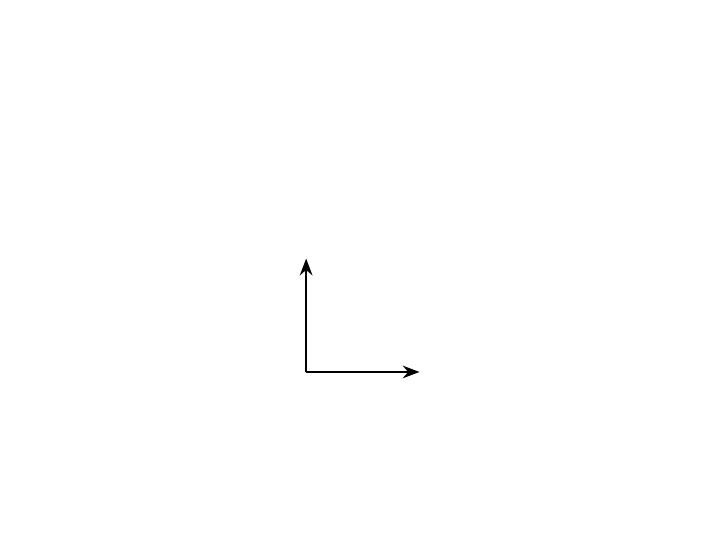Geometric construction of the covariant
derivative
v
u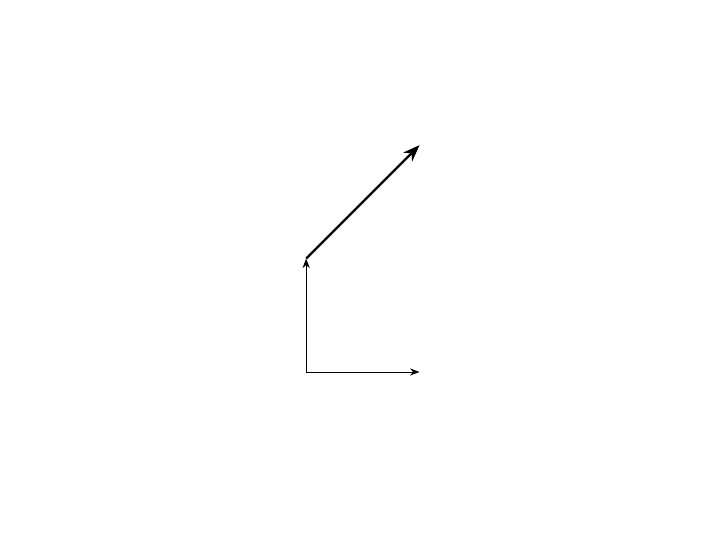Geometric construction of the covariant
derivative
v at the tail of u
u
v at the tip of u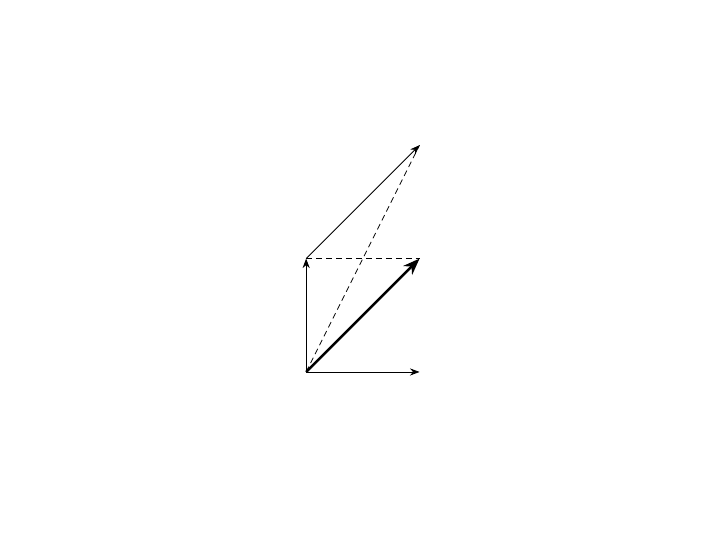Geometric construction of the covariant
derivative
v after parallel transport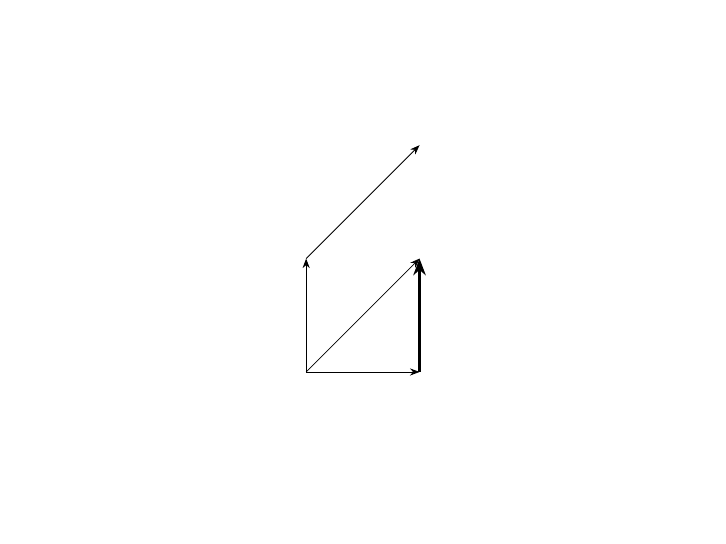Geometric construction of the covariant
derivative
D
u
v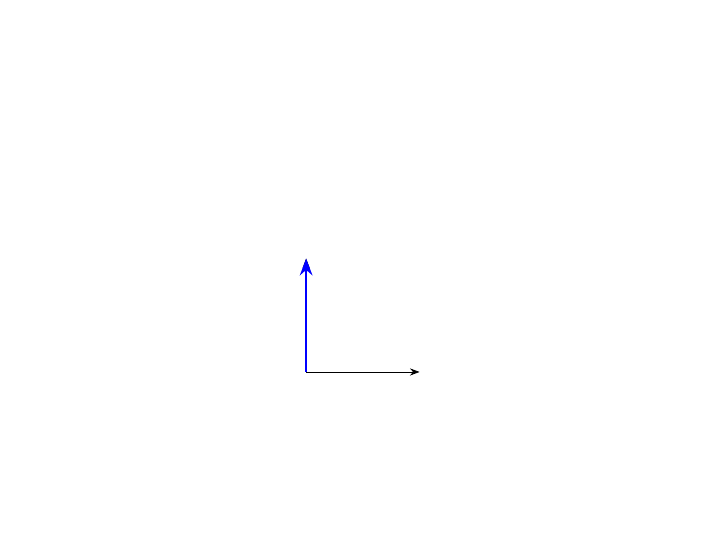Geometric construction of the covariant
derivative
v
u
D
u
vGeodesics
Deﬁnition
The path γ is said to be a geodesic of M if it satisﬁes the
equation
D
˙γ(t)
˙γ(t) = 0.Geodesics
Recall that in local coordinates,
D
˙γ
u =
X
i
du
i
dt
+
X
µ
˙γ
µ
X
j
u
j
A
i
µj
e
i
.
Setting γ(t) = (γ
1
(t), . . . , γ
m
(t)), we have ˙γ(t) =
P
µ
˙γ
µ
(t)
x
µ
,
and setting u(t) = ˙γ(t), we recover the geodesic equations
¨γ
i
=
X
µ
X
j
˙γ
µ
A
i
µj
˙γ
j
,
for i = 1, . . . , m.Connections that are compatible with a
Riemannian metric
Deﬁnition
A connection D on a vector bundle E over the Riemannian
manifold M is compatible with the metric if parallel translation
preserves inner products. That is, if u, u
0
Γ(E) are parallel
sections along γ, then
d
dt
hu
γ(t)
, u
0
γ(t)
i = 0, for t [0, T ].Connections that are compatible with a
Riemannian metric
Lemma
Suppose that D is a connection compatible with the metric. Let
s, u Γ(E) be two arbitrary sections and let γ : [0, T ] M be a
smooth curve. Then,
d
dt
hs, ui = hD
˙γ
s, ui + hs, D
˙γ
ui
along γ(t) for t [0, T ].
In general, if v X(M), then
vhs, ui = hD
v
s, ui + hs, D
v
ui.Connections that are compatible with a
Riemannian metric
Deﬁnition (Symmetric connection)
A connection D on the vector bundle E over the manifold M is
said to be symmetric if it satisﬁes the identity
D
X
Y D
Y
X = [X, Y ],
where [·, ·] is the Lie bracket.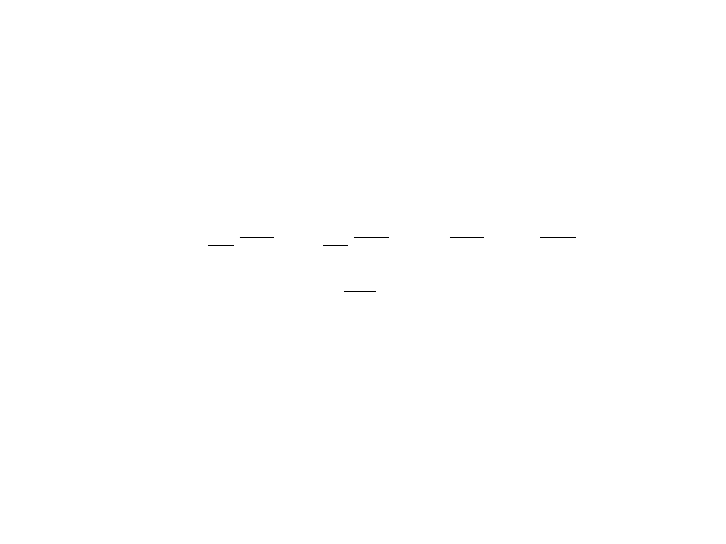Connections that are compatible with a
Riemannian metric
Remark
In local coordinates, we have
0 = D
x
k
x
j
D
x
j
x
k
= A
k
x
j
A
j
x
k
=
X
i
A
i
kj
A
i
jk
x
i
.
Therefore, for all i, j, k {1, . . . , m},
A
i
kj
= A
i
jk
.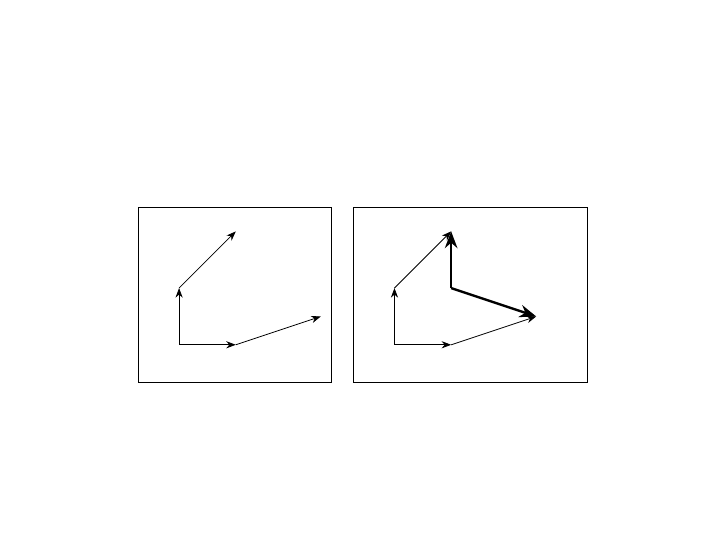Connections that are compatible with a
Riemannian metric
v
t
u
t
u
t+∆t
v
t+∆t
v
t
u
t
u
t+∆t
v
t+∆t
D
u
v
D
v
u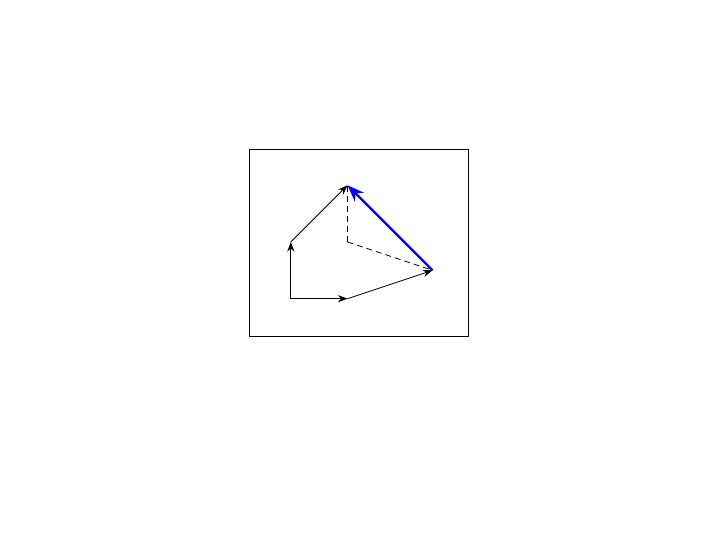Connections that are compatible with a
Riemannian metric
v
t
u
t
u
t+∆t
v
t+∆t
D
u
v D
v
u
Geometric interpretation of the formula D
u
v D
v
u = [u, v]. We
parallel-translate v along u and u along v and then see how much they
fail to commute.Connections that are compatible with a
Riemannian metric
Lemma (Fundamental lemma of Riemannian geometry)
A Riemannian manifold possesses a unique symmetric connection
which is compatible with the metric. This connection is called the
Levi-Civita connection.
The components of the vector potential corresponding to the L-C
connection are the Christoﬀel symbols, given by
Γ
k
ij
=
1
2
P
`
(
i
g
j`
`
g
ij
+
j
g
`i
) g
`k
.Connections that are compatible with a
Riemannian metric
Example
The ﬁrst fundamental form corresponding to the parameterization
of the upper dome of the 2-sphere given by
x(u, v) = u, y(u, v) = v, z(u, v) =
1 u
2
v
2
1/2
,
yields the metric,
g =
v
2
1
u
2
+ v
2
1
du du
uv
u
2
+ v
2
1
du dv
uv
u
2
+ v
2
1
dv du +
u
2
1
u
2
+ v
2
1
dv dvConnections that are compatible with a
Riemannian metric
Example
The corresponding Christoﬀel symbols are:
Γ
u
uu
=
uv
2
u
u
2
+ v
2
1
, Γ
u
uv
=
u
2
v
u
2
+ v
2
1
, Γ
u
vv
=
u
3
u
u
2
+ v
2
1
,
Γ
v
uu
=
v
3
v
u
2
+ v
2
1
, Γ
v
uv
=
uv
2
u
2
+ v
2
1
, Γ
v
vv
=
u
2
1
v
u
2
+ v
2
1
.
The remaining symbols follow from the symmetric nature of the
connection.Outline
1 Basic concepts and tools
2 Connections, covariant diﬀerentiation, and parallel translation
3 CurvatureHolonomy
Deﬁnition (Holonomy along a path)
Again, consider a connection D on a vector bundle E over a
manifold M. Let u E
p
for some point p M and let
γ : [0, T ] M be a path from p to another point q. The
holonomy along the path γ,
H(γ, D): E
p
E
q
,
is deﬁned so that H(γ, D)u E
q
is the result of parallel transport
of u along the direction of ˙γ(t) for t [0, T ].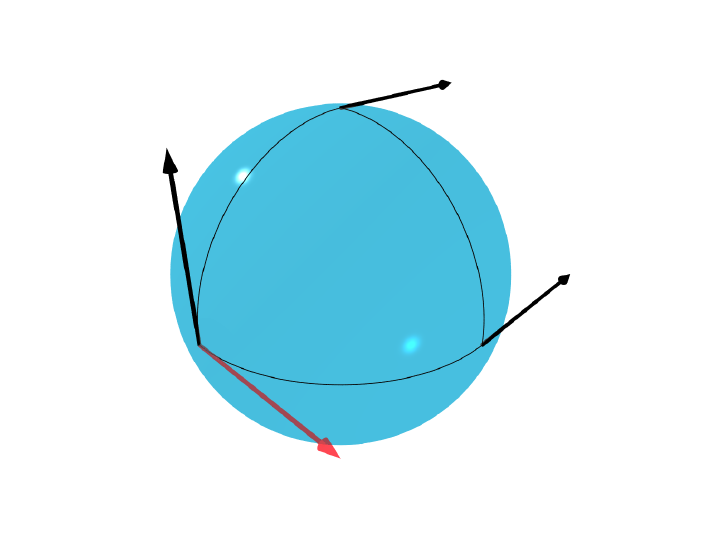Holonomy
Holonomy along a closed path on a sphere.Curvature tensor
The curvature F of a connection D on a vector bundle E over M
measures the failure of covariant derivatives to commute.
In Euclidean space, we have
x
i
x
j
=
x
j
x
i
.
In an arbitrary manifold,
D
x
i
D
x
j
6= D
x
j
D
x
i
,
in general.Curvature tensor
Deﬁnition
Let v, w X(M ) and s Γ(E). We deﬁne the curvature as the
mapping
F (v, w): Γ(E) Γ(E)
s 7→ F (v, w)s =
[D
v
, D
w
] D
[v,w]
sCurvature tensor (local coordinates)
In local coordinates, the Lie bracket of the coordinate ﬁelds
[
µ
,
ν
] vanishes and we obtain the following expression for the
curvature form,
F (µ, ν)e
i
= [D
µ
, D
ν
]e
i
= (D
µ
D
ν
D
ν
D
µ
) e
i
= D
µ
A
j
νi
e
j
D
ν
A
j
µi
e
j
= D
µ
A
j
νi
e
j
D
ν
A
j
µi
e
j
= (
µ
A
j
νi
)e
j
+ A
j
νi
A
k
µj
e
k
(
ν
A
j
µi
)e
j
A
j
µi
A
k
νj
e
k
=
µ
A
j
νi
ν
A
j
µi
+ A
k
νi
A
j
µk
A
k
µi
A
j
νk
e
j
.Curvature tensor (local coordinates)
Therefore,
F
µν
= F
j
µνi
e
j
e
i
where F
j
µνi
=
µ
A
j
νi
ν
A
j
µi
+A
k
νi
A
j
µk
A
k
µi
A
j
νk
.
Or, more succinctly,
F
µν
= (
µ
A
ν
ν
A
µ
+ [A
µ
, A
ν
])
j
i
e
j
e
i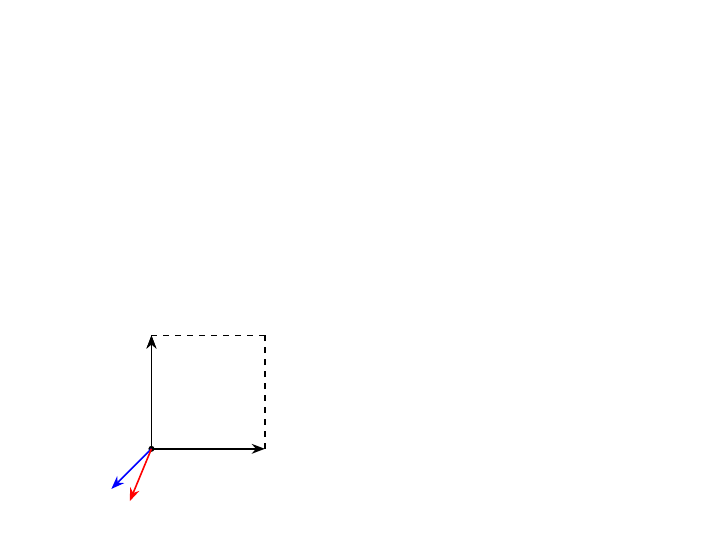Curvature tensor (geometric
interpretation)
Take a vector v E
p
and parallel transport it around a small
square of side ε in the plane (x
µ
, x
ν
). The result is a vector
v
0
E
p
such that
v
0
= (1 F
µν
ε
2
) v.
p
ε
µ
ε
ν
v
v
0
Curvature tells us how much
v
0
fails to be equal to v.